Select Page

# CBSE Maths 12 Science Application of Derivatives MCQ Answers in English

CBSE Maths 12 Science Application of Derivatives MCQ Answers in English to enable students to get Answers in a narrative video format for the specific question.

Expert Teacher provides CBSE Maths 12 Science Application of Derivatives MCQ Answers through Video Answers in English language. This video solution will be useful for students to understand how to write an answer in exam in order to score more marks. This teacher uses a narrative style for a question from Application of Derivatives not only to explain the proper method of answering question, but deriving right answer too.

Please find the question below and view the Answer in a narrative video format.

Question:

## Similar Questions from CBSE, 12th Science, Maths, Application of Derivatives

Question 1 : A cylindrical tank of radius 10 m is being filled with wheat at the rate of the 314 cubic meters per hour. Then the depth of the wheat is increasing at the rate of ___________. (View Answer Video)

Question 2 : The normal at the point (1,1) on the curve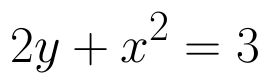is  (View Answer Video)

Question 3 : The slope of the tangent to the curve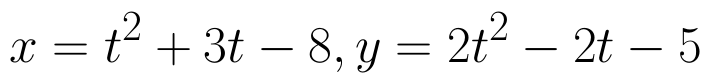at the point (2,-1) is _______________. (View Answer Video)

Question 4 : If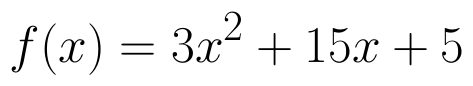, then the approximate value of f (3.02) is  (View Answer Video)

Question 5 : The slope of the tangent to the curve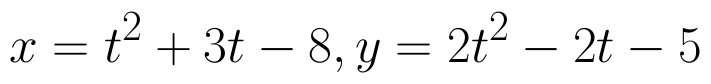at the point (2, -1) is : (View Answer Video)

### Continuity and Differentiability

Question 1 : Differentiate the function w.r.t.x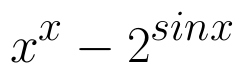. (View Answer Video)

Question 2 : If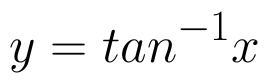, find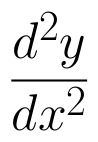in terms of y alone. (View Answer Video)

Question 3 : Findfor the function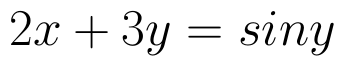. (View Answer Video)

Question 4 : Differentiate the function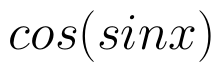with respect to x. (View Answer Video)

Question 5 : Differentiate w.r.t.x the function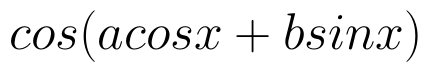, for some constant a and b. (View Answer Video)

### Inverse Trigonometric Functions

Question 1 : Solve for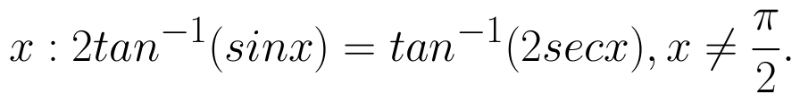(View Answer Video)

Question 2 : Find the value of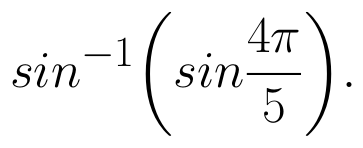What value do you learn from it ? (View Answer Video)

Question 3 :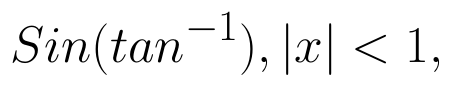is equal to: (View Answer Video)

Question 4 : Solve for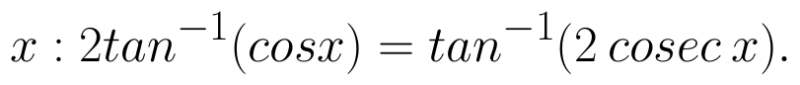(View Answer Video)

Question 5 : Solve for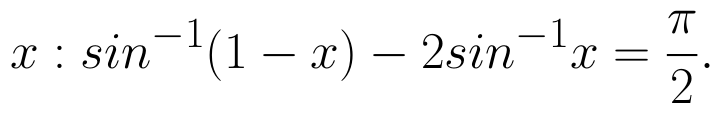(View Answer Video)

### Application of Integrals

Question 1 : Using integration, find the area of the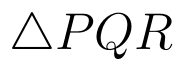co-ordinates whose vertices are P(2,0), Q(4, 5) and R(6,3). (View Answer Video)

Question 2 : Find the area of the circle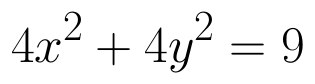which is interior to the parabola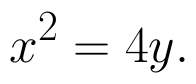(View Answer Video)

Question 3 : Using integration find the area of the triangular region whose sides have the equations y = 2x + 1, y = 3x + 1 and x = 4. (View Answer Video)

Question 4 : Find the area of the region lying in the first quadrant and bounded by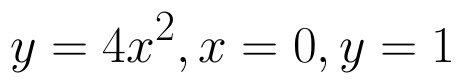and y = 4. (View Answer Video)

Question 5 : Using integration, find the area of the triangle ABC, where A is (2,3), B is (4,7) and C is (6,2). (View Answer Video)